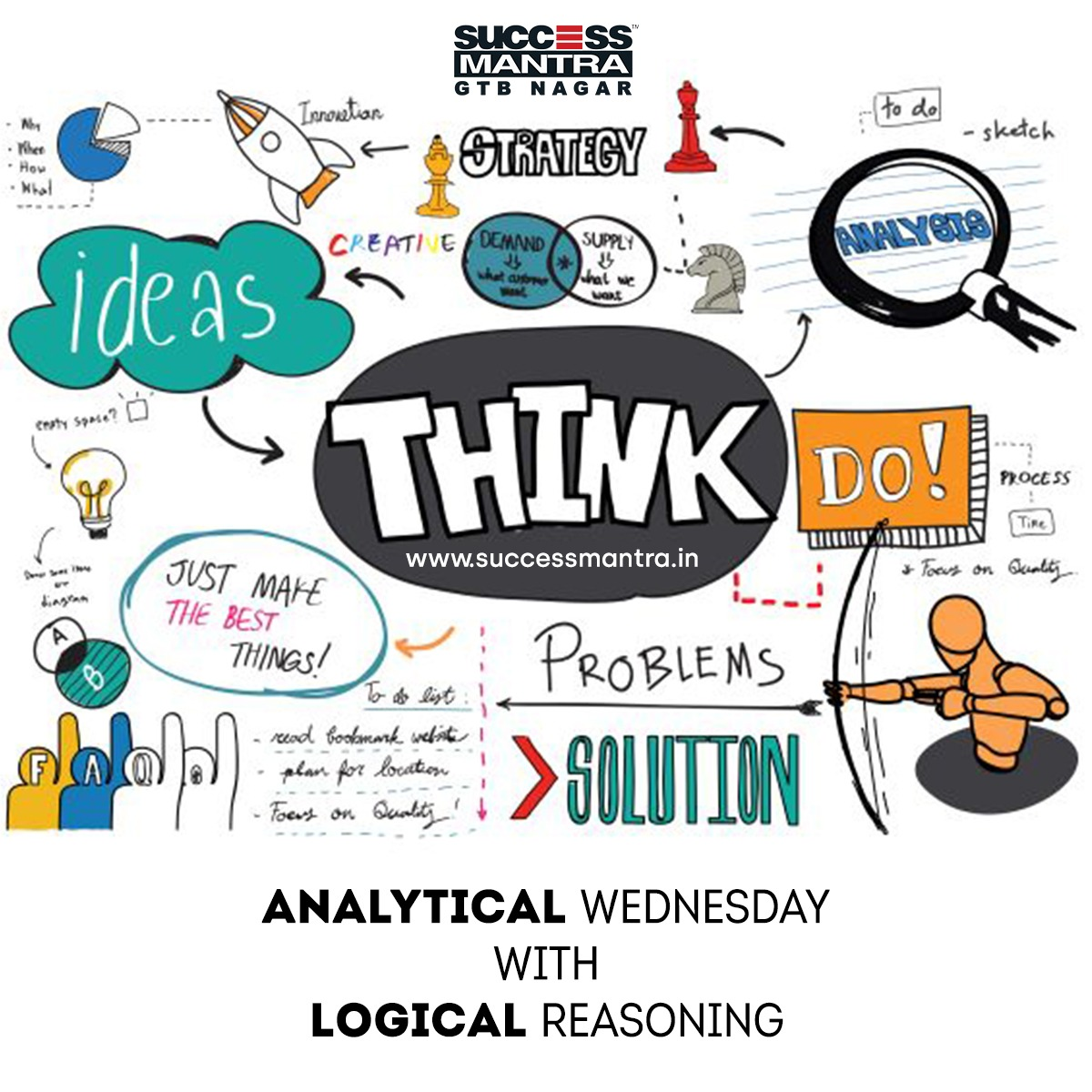## Questions on Logical Reasoning SMLRQ0251. If in a certain code BAT = 23 and CAT = 24, then how will you code HEN = ?

A. 25
B. 35
C. 26
D. 27

2. If A = 26, SUN = 27, Then MOON = ?

A. 52
B. 51
C. 53
D. None of these

3. If A = 2, M = 26, Z = 52, then RAT = ?

A. 70
B. 76
C. 78
D. 39

4. In a certain code, the word DEAL is coded 4 – 5 – 1 – 12 following the same rule of coding, what should be the code of the word GENTS?

A. 7 – 5 – 14 – 20 – 19
B. 6 – 5 – 14 – 20 – 19
C. 5 – 7 – 25 – 19 – 18
D. 7 – 6 – 15 – 21 – 20

5. In certain code DEAF is written as 3587 and FILE is written as 7465. How is IDEAL written in that code?

A. 43568
B. 43586
C. 63548
D. 48536

6. If certain language CHARCOLE coded as 45164913 and MORALE is coded as 296137, how is the word ALLOCHRE coded in that language?

A. 13396875
B. 16693985
C. 13394567
D. 19943785

7. In certain code RAIN is written as 8\$%6 and MORE is written as 7#[email protected] How REMAIN written in that code?

A. #@7\$%6
B. #@&\$%6
C. [email protected]#\$%6
D. [email protected]\$%6